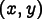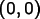# OOF2: The Manual

## Name

Coord — Coordinate classes

## Synopses

### C++ Synopsis

`#include "common/coord.h"`
`class Position {`  virtual Coord position() const = 0;`}`
`class Coord: , public Position {`  Coord();``  Coord(double x,        double y);``  double operator()(int i) const;``  double& operator()(int i);``  Coord& operator+=(const Coord& other);``  Coord& operator-=(const Coord& other);``  Coord& operator*=(double factor);``  Coord& operator/=(double factor);`}`
```Coord operator+(const Coord&, const Coord&)
Coord operator-(const Coord&, const Coord&)
Coord operator*(const Coord&, double)
Coord operator((double, const Coord&)
double cross(const Coord&, const Coord&)
double operator%(const Coord&, const Coord&)
double dot(const Coord&, const Coord&)
double norm2(const Coord&)

bool operator<(const Coord&, const Coord&);
bool operator==(const Coord&, const Coord&);
bool operator!=(const Coord&, const Coord&);
```

### Python Synopsis

`from oof2.SWIG.common import coord`
`class Position:   pass`
`class Coord(Position):`  def __getitem__(self, i)``  def __mul__(self, factor)``  def __rmul__(self, factor)``  def __cmp__(self, other)``

### Source Files

• `SRC/common/coord.C`: C++ code
• `SRC/common/coord.h`: C++ header file
• `SRC/common/coord.swg`: swig code
• `SRC/common/coord.spy`: Python code included in swig output

## Description

`Position` and its subclasses, including `Coord`, represent points on the two dimensional plane of an OOF2 calculation. The other subclass of `Position` is `GaussPoint`, which has a lot more machinery attached to it than `Coord` does. OOF2 extension authors shouldn't have to write code involving `GaussPoints` explicitly, so that class isn't documented here.

`Coord` is a C++ class and is not intended for heavy use in Python. It's swigged so that occasional Python operations can be performed, but most of the arithmetic functions are only available in C++. When there's one `Coord`, there tend to be a lot of them, so if you find yourself doing `Coord` arithmetic in Python, you should think about doing it in C++ instead.

## Methods

### `virtual Coord position() const`

`position` is the only function in the `Position` base class. It effectively converts any `Position` object to a `Coord`. It's useful when a routine needs to operate on generic `Position` objects, but still wants to perform arithmetic with them.

### `Coord(), Coord(double x, double y)`

`Coord(x,y)` creates a `Coord` at position, as you'd expect. The default constructor, `Coord(0)` creates one at.

### `double operator()(int i), __getitem__(i)`

These operators retrieve the x and y components of the `Coord`. The C++ version uses `operator()` instead of `operator[]` for historical reasons, for which we apologize. The argument `i` should be `0` for x and `1` for y.

### `operator+=, operator*, etc.`

The standard arithmetic operations are defined for `Coords` in C++. As noted above, most of them are not defined in Python.

### ```double cross(const Coord&, const Coord&), double operator%(const Coord&, const Coord&)```

The cross product of two `Coords` `a` and `b` can be computed either by `cross(a,b)` or by `a%b`. Because `Coords` are two dimensional vectors, the cross product is a scalar, not a vector. It's equivalent to the z-component of a three dimensional cross product.

### `double dot(const Coord&, const Coord&)`

`dot` returns the dot product of its arguments.

### `double norm2(const Coord&)`

`norm2` returns the square of the norm of its argument. `norm2(x)` is equivalent to `dot(x,x)`.

### `operator<, operator==, operator!=, __cmp__`

The basic comparison operators are available for `Coords` in C++ and Python. The C++ `operator<` and the Python `__cmp__` are provided mostly so that `Coords` can be used as keys in STL maps.

 If we've done our job correctly, that is.

 `GaussPoints`, which are derived from `Positions`, represent special fixed points inside an `Element`, so it doesn't really make sense to do arithmetic with them. Therefore there are no arithmetic operations defined in the `Position` base class.